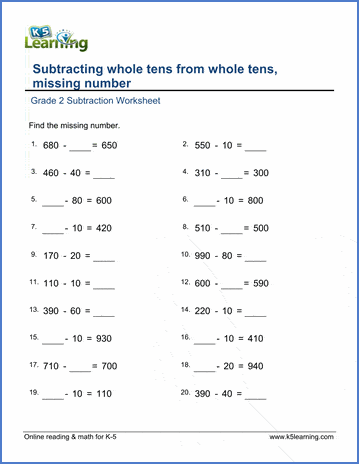# Subtracting whole tens, missing number

## Worksheets: Subtracting whole tens (10-100) from whole tens (100-1,000), missing number

Below are six versions of our grade 2 math worksheet on subtracting whole tens from numbers up to 1,000 ending in a zero.  Students must find the missing number. These worksheets are pdf files.## More subtraction worksheets

Explore all of our subtraction worksheets, from subtracting by counting objects to subtracting large numbers in columns.

## What is K5?

K5 Learning offers reading and math worksheets, workbooks and an online reading and math program for kids in kindergarten to grade 5.  We help your children build good study habits and excel in school.